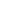# Strategize and apply understanding of the equal sign to solve equivalent expressions.

EngageNY 60 min(s)
In this lesson, students find matching expressions to create true number sentences. They work solely with equations to show and talk about how they would rerepresent a given addition or subtraction problem using an advanced strategy. For example, when given 9 + 6, students decompose the 6 into 1 and 5 and then can add using their new number sentence, 10 + 5 (i.e., 9 + 6 = 10 + 5), using pictures and words.